Latest Banking jobs   »

# Reasoning Ability Quiz For Bank Foundation 2023-20th March

Directions (1-2): In each of the questions below some statements are given followed by some Conclusions. You have to take the given statements to be true even, if they seem to be at variance from commonly known facts. Read all the conclusions and then decide which of the given conclusions logically follows from the given statements disregarding commonly known facts.
(a) If only conclusion I follows.
(b) If only conclusion II follows.
(c) If either conclusion I or II follows.
(d) If neither conclusion I nor II follows.
(e) If both conclusions I and II follow.

Q1. Statements: All honey are sugar
Some sugar is salt
No honey is water
Conclusions: I: Some salt is not water
II: Some honey is water is possibility

Q2. Statements: Some dance are classic
All classic are old
Some old are Gold
Conclusions: I. Some gold are classic
II. No classic is gold

Directions (3-5): In each of the questions below some statements are given followed by some Conclusions. You have to take the given statements to be true even, if they seem to be at variance from commonly known facts. Read all the conclusions and then decide which of the given conclusions logically follows from the given statements disregarding commonly known facts.
(a) If only conclusion I follows.
(b) If only conclusion II follows.
(c) If either conclusion I or II follows.
(d) If neither conclusion I nor II follows.
(e) If both conclusions I and II follow.

Q3. Statements: Only cartoon are Shows
No cartoon is network
Conclusions: I: Some broadband are not cartoon
II: All network can be broadband

Q4. Statements: All car is bike
Only a few bike is truck
All bike is mobile
Conclusions: I: Some Mobile is truck
II: Some car is truck

Q5. Statements: No hydro is water
Some water is Vapour
Some hydro is account
Conclusions: I: All account can never be water
II: Some vapour are not account

Directions (6-10): In each of the questions given below some statements are followed by some conclusions. You have to take the given statements to be true even if they seem to be at variance from commonly known facts. Read all the conclusions and then decide which of the given conclusions logically follows from the given statements disregarding commonly known facts.

Q6. Statements: Some chair are table
Some Running are Jogging
Some Jogging are Fire
No table is Jogging
Conclusion: I. Some running are Fire
II. No Fire are running
III. Some Jogging are chair
(a) Only I is true
(b) Only II is true
(c) Either I or II is true
(d) Only III is true
(e) All is true

Q7. Statements: Only Brown is Pink
Some Brown is white.
All white are clay
Conclusion: I. Some white can be Pink
II. Some clay can be Brown
III. All Brown can be Clay
(a) Only II is true
(b) Both II and III is true
(c) Only I is true
(d) None follows
(e) Either II or III is true

Q8. Statements: All Font are letter
No letter are Finger
No Font is Hand
Conclusion: I. Some letter are not Hand.
II. No Font is Finger
III. Some hand are not Finger
(a) Both II and III is true
(b) Only III is true
(c) Both I and II is true
(d) Both I and III is true
(e) Only I is true

Q9. Statements: Some Nature are colour.
Some colour is price
Conclusions: I. Some price are nature
III. No nature is price.
(a) Only I follows
(b) Only II follows
(c) Either I or III follow
(d) Only III follows
(e) None follows

Q10. Statements: Some time are work
No work is wages
Only speed is wages
Conclusions: I. Some work is speed
II. Some speed is not work
III. Some time is not Wages
(a) Only I follows
(b) Only II and III follow
(c) Only I and III follow
(d) Only I and II follow
(e) None of these

Directions (11-15): The following questions are based on the five three digits number given below.

451  685  254  723  132

Q11. Which of the following numbers will be obtained if the second digit of the greatest number is subtracted from the second digit of the lowest number after adding one to each of the numbers?
(a) 1
(b) 2
(c) 3
(d) 4
(e) 5

Q12. If in each number, the first and the last digits are interchanged then, which of the following thus formed will be the third highest number?
(a) 451
(b) 685
(c) 254
(d) 723
(e) 132

Q13. If in each number, the second and the third digits are interchanged then, which will be the second highest number?
(a) 451
(b) 685
(c) 254
(d) 723
(e) 132

Q14. If one is subtracted from the first digit of each of the numbers and then the first and the third digits are interchanged, which of the following will be the lowest?
(a) 451
(b) 685
(c) 254
(d) 723
(e) 132

Q15. If in each number, all the three digits are arranged in the ascending order within the number, which of the following will be the third lowest number?
(a) 451
(b) 685
(c) 254
(d) 723
(e) 132

Solutions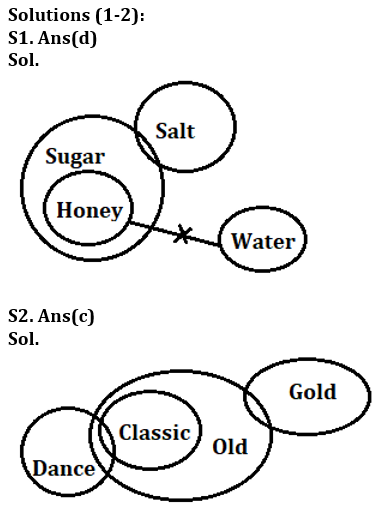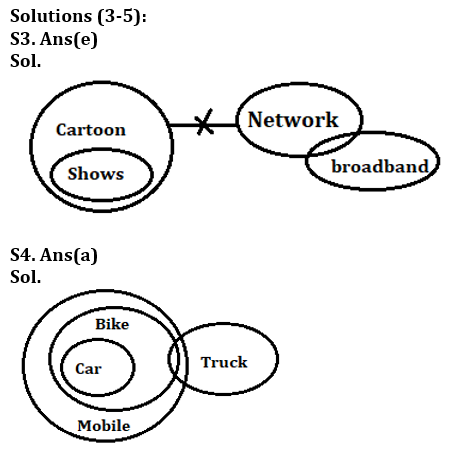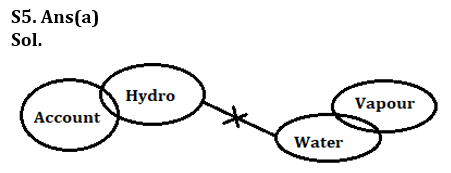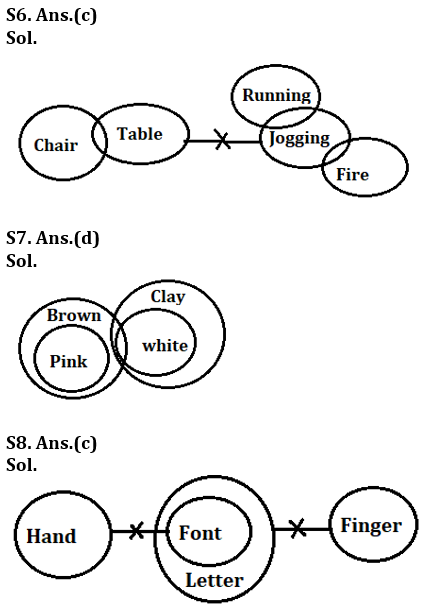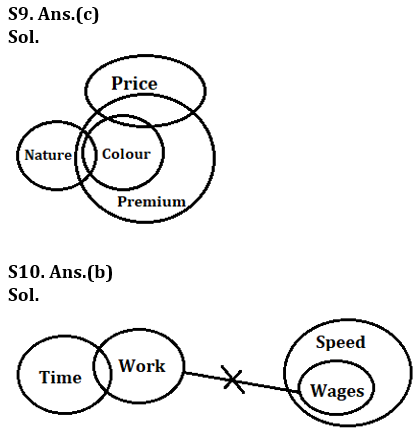Solutions (11-15):
S11. Ans.(a)
Sol. 3-2=1
S12. Ans.(d)
S13. Ans.(b)
S14. Ans.(a)
S15. Ans.(d)## FAQs

### What is the selection process of the Bank Clerk?

The selection process of the Bank Clerk is Prelims & Mains.

#### Congratulations!Union Budget 2023-24: Free PDF